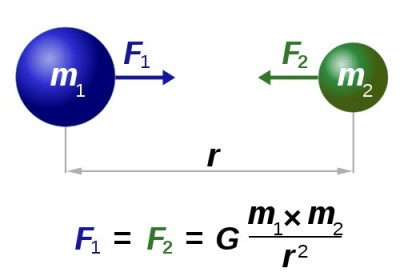# Universal Law of GravitationContent:

• Who Discovered the Law of Gravity?
• Law of Universal Gravitation Definition
• Universal Gravitation Equation
• Gravitational Theory and Weightlessness in Space
• Universal Law of Gravitation, Video

## Who Discovered the Law of Gravity?

It is not a secret for anyone that the law of universal gravitation was discovered by the great English scientist Isaac Newton. According to legend, he was walking in the garden. At that moment, an apple fell from the tree (in one version it fell directly on the head of a physicist, in another it simply fell). Later this apple became famous Newton’s apple. An apple that fell on Newton’s head inspired the discovery of the law of universal gravitation because the Moon in the night sky was not falling, but the apple fell. The scientist probably thought that some force acts on the Moon (causing it to rotate in orbit), and some force acts on the apple, causing it to fall to the ground.

This whole story about an apple is just a beautiful myth about how did Isaac Newton discover gravity. In fact, an apple fell or not, it is not so important. It is important that the scientist discovered and formulated the law of universal gravitation, which is now one of the cornerstones of both physics and astronomy.

Long before Newton, people observed both things falling on the ground and stars in the sky. Before him, they believed that there are two types of gravity: terrestrial (acting exclusively within the Earth, causing bodies to fall) and celestial (acting on stars and Moon). Newton was the first to unite these two types of gravity in his head, the first to understand that gravity is only one and its action can be described by a universal physical law.

## Law of Universal Gravitation Definition

What causes gravity? According to the universal law of gravitation, all material bodies attract each other, while the attractive force does not depend on the physical or chemical properties of the bodies. It depends only on the weight of the bodies and the distance between them (this is the simplest explanation). Besides this, it is necessary to take into account the fact that all bodies on Earth are affected by the force of gravity of our planet itself, which is called gravity (from Latin – gravitas).

Let us now try to formulate and write down the universal law of gravitation: the force of gravity between two objects with masses m1 and m2 and separated by distance R is directly proportional to both masses and inversely proportional to the square of the distance between them.

## Universal Gravitation EquationG in this formula is the gravitational constant, equal to 6,67408(31)•10−11. This is the impact on any material object of the gravitational force of our planet.

F in this formula is the force of gravity. So now you know how to find the force of gravity.

## Gravitational Theory and Weightlessness in Space

The law of universal gravitation formed the basis of celestial mechanics and astronomy. Due to it, we can explain the nature of the motion of celestial bodies, as well as the phenomenon of weightlessness. Being in outer space at a considerable distance from the gravitational force of such a large body like a planet, any material object (for example, a spacecraft with astronauts on board) will be in a state of weightlessness. Since the force of gravity of the Earth (G in the gravity equation) or some other planet will no longer affect it.